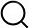# Also in the Article

Predicted friction coefficient

Procedure

The ratio between wet and dry friction coefficients was calculated on the basis of the assumption that the contribution of dry-on-dry contacts to the wet friction coefficient was negligible. Shear stress in the liquid is equal to shear stress in the material. Thus, $τ=FA=μFNA=ηUh$. This way, we found a relation for the friction coefficient$μ=ηUAhFN$(3)where η is the estimated viscosity, U is the sliding speed, A is the load-bearing area, h is the average thickness of the liquid, and FN is the normal force.

As the viscosity was estimated on the basis of ΛI and to compare data with the measurements in Fig. 3B, we plot the predicted ratio of dry and wet friction coefficients as a function of ΛI (see fig. S6).

Q&A